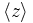# Double cover of symmetric group

## Definition

The term double cover of symmetric group is used for a stem extension where the base normal subgroup is cyclic group:Z2 and the quotient group is an symmetric group of finite degree.

A double cover exists for the symmetric group$S_n$ only when$n \ge 4$. Further, for each$n$, there are two possibilities for the double cover, the "+" type and the "-" type.

If we consider the group cohomology of symmetric groups and in particular the second cohomology group for trivial group action$H^2(S_n;\mathbb{Z}_2)$ for$n \ge 4$, this second cohomology group is isomorphic to the Klein four-group, and two of the three nontrivial elements of the group correspond to these double covers. The third nontrivial element corresponds to a group extension where the alternating group in the quotient is kept intact and the odd permutations square to the non-identity central element.

In all cases, both double covers are Schur covering groups for the corresponding symmetric group, because the Schur multiplier is precisely cyclic group:Z2.

### Presentation for "-" type double cover

This group, denoted$2 \cdot S_n^-$, has a presentation with generating set of size$n$ given by$z,s_1,s_2,\dots,s_{n-1}$. The idea is that under the surjective map to$S_n$,$z$ maps to the identity and the relations collapse to the Coxeter presentation of$S_n$. The subgroup$\langle z \rangle$ is the base cyclic group:Z2. The relations (here$e$ denotes the identity element):

Relation Condition on subscripts Number of such relations What it descends to when we quotient to$S_n$$\! z^2 = e$ no subscripts 1$e = e$, i.e., a vacuous relation$\!s_i^2 = z$$1 \le i \le n - 1$$n - 1$$s_i^2 = e$$\! s_{i+1}s_is_{i+1} = s_is_{i+1}s_iz$$1 \le i \le n - 2$$n - 2$$s_{i+1}s_is_{i+1} = s_is_{i+1}s_i$$\! s_js_i = s_is_jz$$1 \le i < j \le n - 1$ and$|i - j| \ge 2$$(n - 2)(n - 3)/2$$s_js_i = s_is_j$
Total (--) --$(n^2 - n + 2)/2$ --

### Presentation for "+" type double cover

This group, denoted$2 \cdot S_n^+$, has a presentation with generating set of size$n$ given by$z,s_1,s_2,\dots,s_{n-1}$. The idea is that under the surjective map to$S_n$,$z$ maps to the identity and the relations collapse to the Coxeter presentation of$S_n$. The subgroup$\langle z \rangle$ is the base cyclic group:Z2. The relations (here$e$ denotes the identity element):

Relation Condition on subscripts Number of such relations What it descends to when we quotient to$S_n$$\! z^2 = e$ no subscripts 1$e = e$, i.e., a vacuous relation$\! s_iz = zs_i$$1 \le i \le n- 1$$n - 1$$s_i = s_i$, i.e., a vacuous relation$\! s_i^2 = e$$1 \le i \le n - 1$$n - 1$$s_i^2 = e$$\!s_{i+1}s_is_{i+1} = s_is_{i+1}s_i$$1 \le i \le n - 2$$n - 2$$s_{i+1}s_is_{i+1} = s_is_{i+1}s_i$$\! s_js_i = s_is_jz$$1 \le i < j \le n - 1$ and$|i - j| \ge 2$$(n - 2)(n - 3)/2$$s_js_i = s_is_j$
Total (--) --$(n^2 + n)/2$ --

## Particular cases$n$ order of symmetric group$S_n$ equals$n!$ order of double cover of symmetric group =$2 (n!)$ (twice the preceding column) symmetric group$S_n$$2 \cdot S_n^-$ (double cover of "-" type)$2 \cdot S_n^+$ (double cover of "+" type) Cohomology information Cohomology group information
4 24 48 symmetric group:S4 binary octahedral group general linear group:GL(2,3) group cohomology of symmetric group:S4 second cohomology group for trivial group action of S4 on Z2
5 120 240 symmetric group:S5 double cover of symmetric group:S5 of minus type double cover of symmetric group:S5 of plus type group cohomology of symmetric group:S5 second cohomology group for trivial group action of S5 on Z2